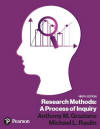﻿ Degrees of Freedom (df)Graziano & Raulin
Research Methods (9th edition)

## Degrees of Freedom

Degrees of freedom, a basic statistical concept needed in many statistical computations, refers to the number of scores that are free to vary.

Suppose that you are asked to pick any three numbers. There are no restrictions, and the numbers are completely free to vary. In standard terminology, there would be three degrees of freedom; that is, three numbers are free to vary.

Now suppose that you are to choose any three numbers, but they must total 15; now there is one restriction on the numbers. Because of the restriction, you will lose some of the freedom to vary the three numbers that you choose. If you choose the numbers 5 and 1 as the first two numbers, you must choose 9 as the third number to arrive at the total of 15. If you choose the numbers 8 and 11 as the first two numbers, the third must be -4 in order to total 15. In both examples, two numbers are free to vary, but one is not. In standard terminology, there are two degrees of freedom; that is, two numbers are free to vary. In comparison to the first example, in which there was no restriction and all the scores could be freely selected, you have lost a degree of freedom.

Now suppose that you are to choose three scores in which (1) the total must be 15 and (2) the first score must be 7. Note that there are two restrictions placed on these scores. Consequently, two degrees of freedom have been lost, leaving only one degree of freedom. The only score that can vary freely is the second score.

In statistics, the restrictions imposed on data are not arbitrary as they were in this example. Instead, they are determined by the demands of the statistical procedures. Many statistical procedures require that values, such as the population mean, be estimated. These estimates constitute restrictions. The more such restrictions there are, the more degrees of freedom that are lost. In the computation of the variance, one such restriction is imposed and, consequently, the degrees of freedom are reduced by one. Hence, the denominator is N - 1.

 Use the Back Arrow Key on the Browser Program to Return